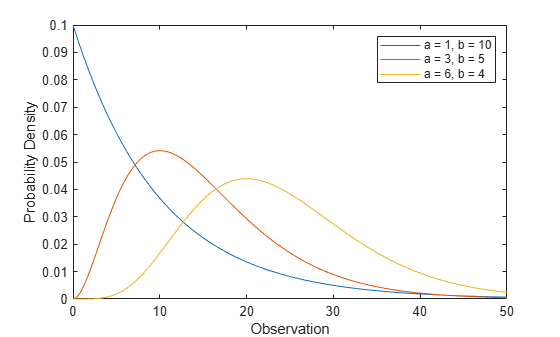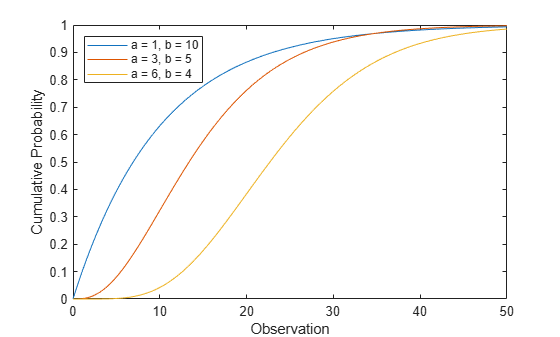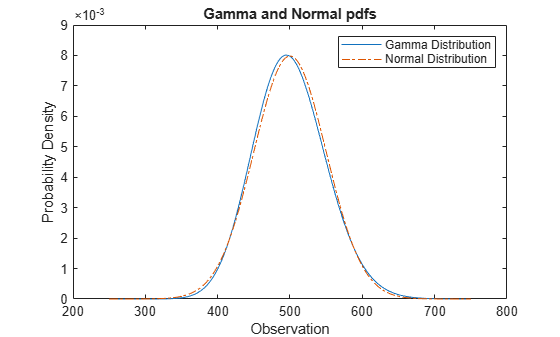## Gamma Distribution

### Overview

The gamma distribution is a two-parameter family of curves. The gamma distribution models sums of exponentially distributed random variables and generalizes both the chi-square and exponential distributions.

Statistics and Machine Learning Toolbox™ offers several ways to work with the gamma distribution.

### Parameters

The gamma distribution uses the following parameters.

ParameterDescriptionSupport
`a` Shapea` > 0`
`b`Scaleb` > 0`

The standard gamma distribution has unit scale.

The sum of two gamma random variables with shape parameters a1 and a2 both with scale parameter b is a gamma random variable with shape parameter a = a1 + a2 and scale parameter b.

#### Parameter Estimation

The likelihood function is the probability density function (pdf) viewed as a function of the parameters. The maximum likelihood estimates (MLEs) are the parameter estimates that maximize the likelihood function for fixed values of `x`.

The maximum likelihood estimators of a and b for the gamma distribution are the solutions to the simultaneous equations

`$\begin{array}{c}\mathrm{log}\stackrel{^}{a}-\psi \left(\stackrel{^}{a}\right)=\mathrm{log}\left(\overline{x}/{\left(\prod _{i=1}^{n}{x}_{i}\right)}^{1/n}\right)\\ \stackrel{^}{b}=\frac{\overline{x}}{\stackrel{^}{a}}\end{array}$`

where $\overline{x}$ is the sample mean for the sample x1, x2, …, xn, and Ψ is the digamma function `psi`.

To fit the gamma distribution to data and find parameter estimates, use `gamfit`, `fitdist`, or `mle`. Unlike `gamfit` and `mle`, which return parameter estimates, `fitdist` returns the fitted probability distribution object `GammaDistribution`. The object properties `a` and `b` store the parameter estimates.

For an example, see Fit Gamma Distribution to Data.

### Probability Density Function

The pdf of the gamma distribution is

`$y=f\left(x|a,b\right)=\frac{1}{{b}^{a}\Gamma \left(a\right)}{x}^{a-1}{e}^{\frac{-x}{b}},$`

where Γ( · ) is the Gamma function.

For an example, see Compute Gamma Distribution pdf.

### Cumulative Distribution Function

The cumulative distribution function (cdf) of the gamma distribution is

`$p=F\left(x|a,b\right)=\frac{1}{{b}^{a}\Gamma \left(a\right)}\underset{0}{\overset{x}{\int }}{t}^{a-1}{e}^{\frac{-t}{b}}dt.$`

The result p is the probability that a single observation from the gamma distribution with parameters a and b falls in the interval [0 x].

For an example, see Compute Gamma Distribution cdf.

The gamma cdf is related to the incomplete gamma function `gammainc` by

`$f\left(x|a,b\right)=\text{gammainc}\left(\frac{x}{b},a\right).$`

### Inverse Cumulative Distribution Function

The inverse cumulative distribution function (icdf) of the gamma distribution in terms of the gamma cdf is

`$x={F}^{-1}\left(p|a,b\right)=\left\{x:F\left(x|a,b\right)=p\right\},$`

where

`$p=F\left(x|a,b\right)=\frac{1}{{b}^{a}\Gamma \left(a\right)}\underset{0}{\overset{x}{\int }}{t}^{a-1}{e}^{\frac{-t}{b}}dt.$`

The result x is the value such that an observation from the gamma distribution with parameters a and b falls in the range [0 x] with probability p.

The preceding integral equation has no known analytical solution. `gaminv` uses an iterative approach (Newton's method) to converge on the solution.

### Descriptive Statistics

The mean of the gamma distribution is ab.

The variance of the gamma distribution is ab2.

### Examples

#### Fit Gamma Distribution to Data

Generate a sample of `100` gamma random numbers with shape `3` and scale `5`.

`x = gamrnd(3,5,100,1);`

Fit a gamma distribution to data using `fitdist`.

`pd = fitdist(x,'gamma')`
```pd = GammaDistribution Gamma distribution a = 2.7783 [2.1374, 3.61137] b = 5.73438 [4.30198, 7.64372] ```

`fitdist` returns a `GammaDistribution` object. The intervals next to the parameter estimates are the 95% confidence intervals for the distribution parameters.

Estimate the parameters `a` and `b` using the distribution functions.

`[muhat,muci] = gamfit(x) % Distribution specific function`
```muhat = 1×2 2.7783 5.7344 ```
```muci = 2×2 2.1374 4.3020 3.6114 7.6437 ```
`[muhat2,muci2] = mle(x,'distribution','gamma') % Generic function`
```muhat2 = 1×2 2.7783 5.7344 ```
```muci2 = 2×2 2.1374 4.3020 3.6114 7.6437 ```

#### Compute Gamma Distribution pdf

Compute the pdfs of the gamma distribution with several shape and scale parameters.

```x = 0:0.1:50; y1 = gampdf(x,1,10); y2 = gampdf(x,3,5); y3 = gampdf(x,6,4);```

Plot the pdfs.

```figure; plot(x,y1) hold on plot(x,y2) plot(x,y3) hold off xlabel('Observation') ylabel('Probability Density') legend('a = 1, b = 10','a = 3, b = 5','a = 6, b = 4')```#### Compute Gamma Distribution cdf

Compute the cdfs of the gamma distribution with several shape and scale parameters.

```x = 0:0.1:50; y1 = gamcdf(x,1,10); y2 = gamcdf(x,3,5); y3 = gamcdf(x,6,4);```

Plot the cdfs.

```figure; plot(x,y1) hold on plot(x,y2) plot(x,y3) hold off xlabel('Observation') ylabel('Cumulative Probability') legend('a = 1, b = 10','a = 3, b = 5','a = 6, b = 4',"Location","northwest")```#### Compare Gamma and Normal Distribution pdfs

The gamma distribution has the shape parameter $\mathit{a}$ and the scale parameter $\mathit{b}$. For a large $\mathit{a}$, the gamma distribution closely approximates the normal distribution with mean $\mu =\mathit{ab}$ and variance ${\sigma }^{2}=\mathit{a}{\mathit{b}}^{2}$.

Compute the pdf of a gamma distribution with parameters `a = 100` and `b = 5`.

```a = 100; b = 5; x = 250:750; y_gam = gampdf(x,a,b);```

For comparison, compute the mean, standard deviation, and pdf of the normal distribution that gamma approximates.

`mu = a*b`
```mu = 500 ```
`sigma = sqrt(a*b^2)`
```sigma = 50 ```
`y_norm = normpdf(x,mu,sigma);`

Plot the pdfs of the gamma distribution and the normal distribution on the same figure.

```plot(x,y_gam,'-',x,y_norm,'-.') title('Gamma and Normal pdfs') xlabel('Observation') ylabel('Probability Density') legend('Gamma Distribution','Normal Distribution')```The pdf of the normal distribution approximates the pdf of the gamma distribution.

### Related Distributions

• Beta Distribution — The beta distribution is a two-parameter continuous distribution that has parameters a (first shape parameter) and b (second shape parameter). If X1 and X2 have standard gamma distributions with shape parameters a1 and a2 respectively, then $Y=\frac{{X}_{1}}{{X}_{1}\text{​}+{X}_{2}}$ has a beta distribution with shape parameters a1 and a2.

• Chi-Square Distribution — The chi-square distribution is a one-parameter continuous distribution that has parameter ν (degrees of freedom). The chi-square distribution is equal to the gamma distribution with 2a = ν and b = 2.

• Exponential Distribution — The exponential distribution is a one-parameter continuous distribution that has parameter μ (mean). The exponential distribution is equal to the gamma distribution with a = 1 and b = μ. The sum of k exponentially distributed random variables with mean μ is the gamma distribution with parameters a = k and μ = b.

• Nakagami Distribution — The Nakagami distribution is a two-parameter continuous distribution with shape parameter µ and scale parameter ω. If x has a Nakagami distribution, then x2 has a gamma distribution with a = μ and ab = ω.

• Normal Distribution — The normal distribution is a two-parameter continuous distribution that has parameters μ (mean) and σ (standard deviation). When a is large, the gamma distribution closely approximates a normal distribution with μ = ab and σ2 = ab2. For an example, see Compare Gamma and Normal Distribution pdfs.

 Abramowitz, Milton, and Irene A. Stegun, eds. Handbook of Mathematical Functions: With Formulas, Graphs, and Mathematical Tables. 9. Dover print.; [Nachdr. der Ausg. von 1972]. Dover Books on Mathematics. New York, NY: Dover Publ, 2013.

 Evans, Merran, Nicholas Hastings, and Brian Peacock. Statistical Distributions. 2nd ed. New York: J. Wiley, 1993.

 Hahn, Gerald J., and Samuel S. Shapiro. Statistical Models in Engineering. Wiley Classics Library. New York: Wiley, 1994.

 Lawless, Jerald F. Statistical Models and Methods for Lifetime Data. 2nd ed. Wiley Series in Probability and Statistics. Hoboken, N.J: Wiley-Interscience, 2003.

 Meeker, William Q., and Luis A. Escobar. Statistical Methods for Reliability Data. Wiley Series in Probability and Statistics. Applied Probability and Statistics Section. New York: Wiley, 1998.

 Marsaglia, George, and Wai Wan Tsang. “A Simple Method for Generating Gamma Variables.” ACM Transactions on Mathematical Software 26, no. 3 (September 1, 2000): 363–72. https://doi.org/10.1007/978-1-4613-8643-8.